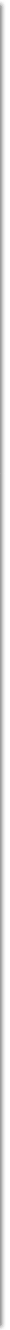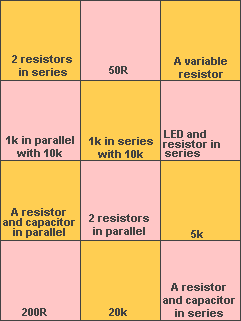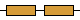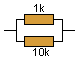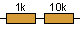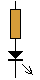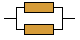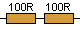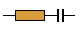Correct.
Click on a component, pull the circuit apart, and try again.

BASIC
ELECTRONICS COURSE
Page 2Question 28: How can you make a 292 ohm resistor?

Ans: This is an unusual request but two resistors from the "standard range" of resistors can be placed in series and the final resistance is the ADDITION of the two values. You will learn more about this later but the two standard  values to use are 220 ohm and 68 ohm, making a total of 220 + 68 = 288 ohm.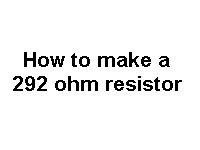To see the pages on RESISTORS IN SERIES - you need to subscribe

Question 29: How do you connect resistors in parallel?

Ans: See animation: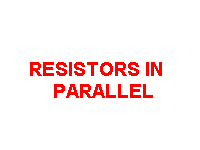Question 29a: Can you make a 292 ohm resistor with resistors in parallel?

Ans: Yes you can, but the maths is very complex.

To see the pages on RESISTORS IN PARALLEL - you need to subscribe
TEST:

Drag the components to the correct position on the map. Click to release:

Question 30: The resistor is a common component in an electronic circuit. Name another common component.

Ans: the capacitor.

There are a number of different types of capacitor and here are 4 from our Library of Symbols: (you need to subscribe to access the Library)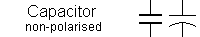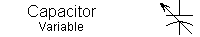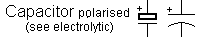Question 31: How does a capacitor work?

Ans: When a voltage is connected to a capacitor it charges up. It's a bit like charging the battery in your mobile phone. In fact the mobile phone battery  can be considered to be a very large capacitor.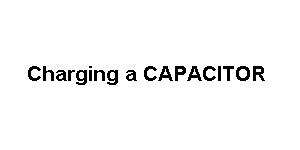Question 32: Does a capacitor "fill up" (charge) at a constant rate?

Ans: No. A fully discharged capacitor fills up (charges) at a fast rate at the beginning of its charge-cycle and and fills-up at a slower and slower rate. It's a bit like filling a car with petrol. The driver fills up quickly at the beginning and as he know the tank is getting full, he slows down the flow of petrol. With a capacitor, this charge-rate is a natural phenomena.

Question 33: Why does a capacitor "fill-up" at a slower and slower rate?

Ans:  It's to do with the voltage of the supply that is charging the capacitor.
Another name for voltage is "pressure." When a capacitor is uncharged, it
has zero voltage across it (in it) and the pressure of the supply voltage will allow a high current to flow and this will begin to charge the capacitor very quickly.
As it gets charged, a voltage builds up across the capacitor and this opposes the voltage of the supply. The result is the supply voltage sees and opposing voltage and thus it does not have as much "pressure" as before to deliver the current into the capacitor and the "rate of charge" decreases.  The "time-lapse animation below shows how the capacitor fills up quickly at the beginning and the filling process slows down.
The graph shows the voltage across the capacitor does not rise in a linear (straight line).
All you have to remember is the fact that a capacitor does not charge in a linear fashion but if you consider the first part of the charging curve, the rise is fairly linear!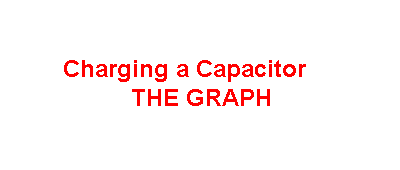Question 34: Can we charge a capacitor then connect the capacitor to a LED?

Ans: Yes. The LED will illuminate brightly then gradually dim as shown in the animation: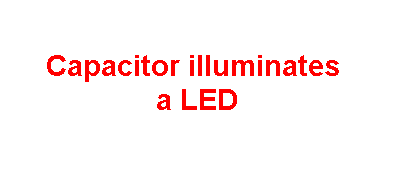WHAT HAVE WE COVERED?

We have shown how to connect a LED to a supply (a supply voltage) and turn it on by selecting a suitable current limiting resistor to give the required illumination.
The resistor can be a single resistor or two resistors in series. We have shown that the total resistance of two resistors in series is the ADDITION of their resistance values. The LED must be connected to a supply around the correct way, with the anode lead to the positive rail and the cathode lead to the negative rail, via a current limiting resistor. The voltage that develops across a LED when it is operating is called the "characteristic voltage" and for a red LED this is 1.7v. The maximum current for a LED is 25mA and the correct current limiting resistor value can be worked out  using Ohms Law.
We have shown how a capacitor charges when connected to a supply voltage. The charge-rate is not linear but that will be explained in more detail later. A capacitor is like a rechargeable battery. It can be charged and its energy used to operate a device.